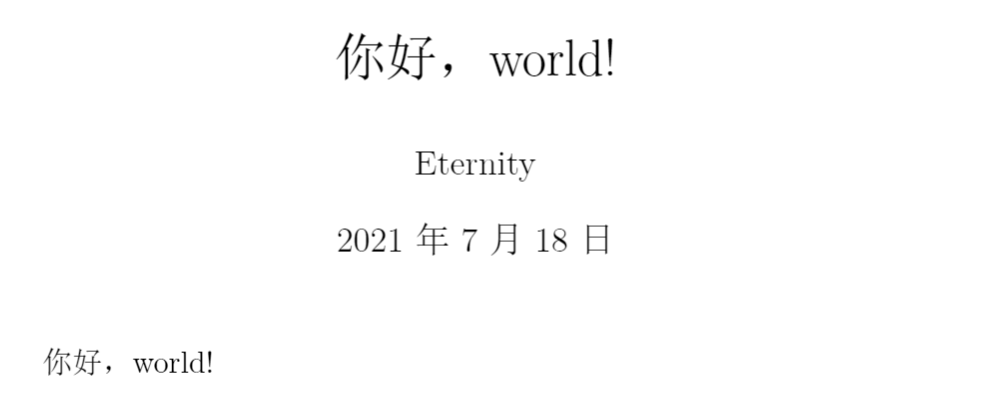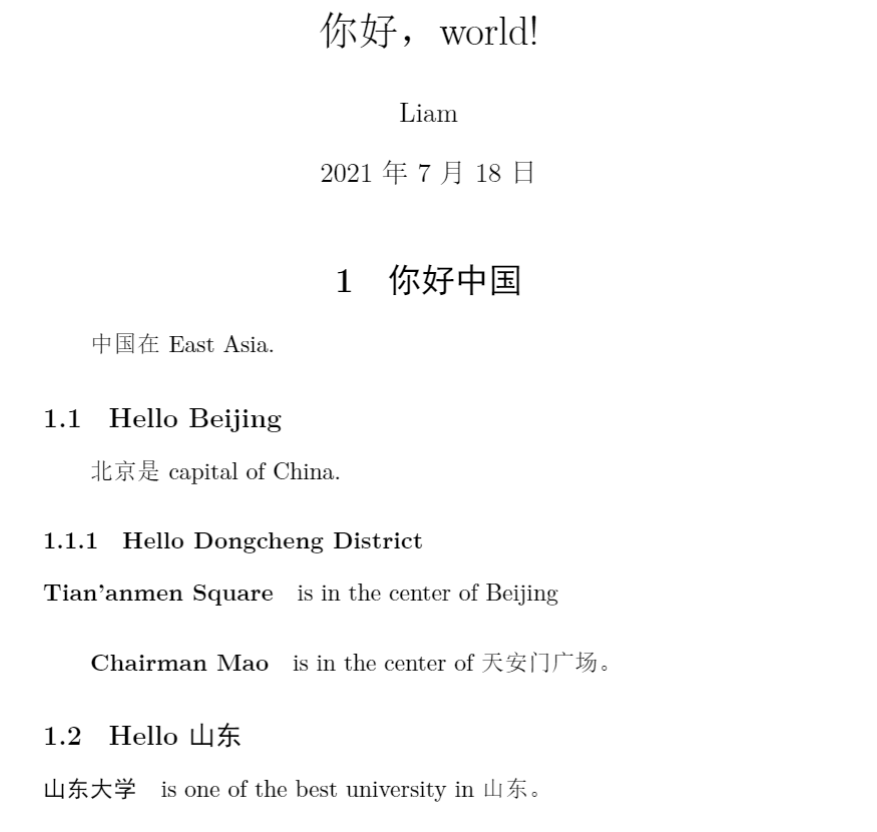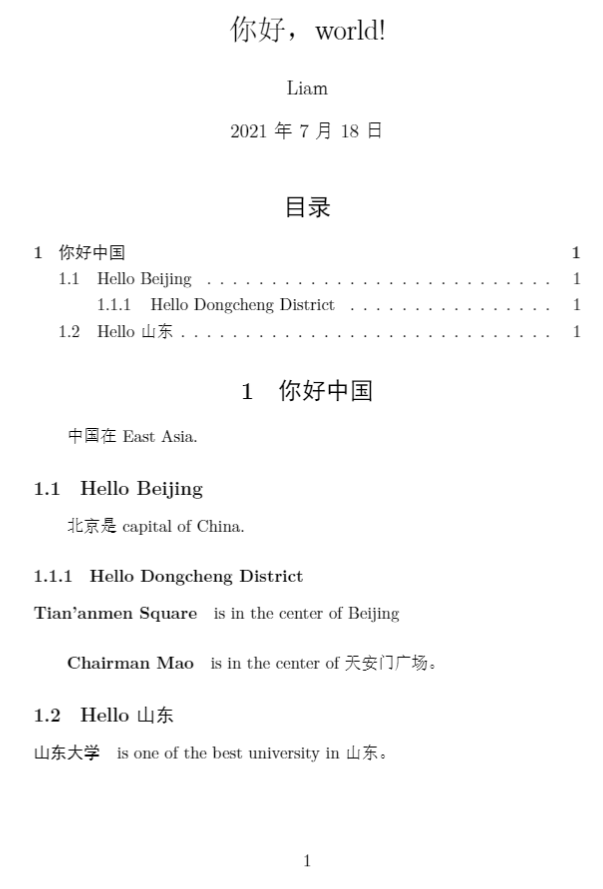LaTex环境配置和基本功能模块

## 基本文档和中英文

### 基本文档

\documentclass{article}
% 这里是导言区
\begin{document}
Hello, world!
\end{document}


Tex以%作为注释标记。若要输出百分号%字符本身，则要在前面加上反斜杠\进行转义（escape）。

### 中英文混排

\documentclass[UTF8]{ctexart}
\begin{document}

\end{document}


### 组织文章基本内容

1. 标题、作者、日期
\documentclass[UTF8]{ctexart}
\title{你好，world!}
\author{Eternity}
\date{\today}
\begin{document}
\maketitle

\end{document}1. 章节和段落
\documentclass[UTF8]{ctexart}
\title{你好，world!}
\author{Liam}
\date{\today}
\begin{document}
\maketitle
\section{你好中国}

\subsection{Hello Beijing}

\subsubsection{Hello Dongcheng District}
\paragraph{Tian'anmen Square}
is in the center of Beijing
\subparagraph{Chairman Mao}
is in the center of 天安门广场。
\subsection{Hello 山东}
\paragraph{山东大学} is one of the best university in 山东。
\end{document}


• \section{·}
• \subsection{·}
• \subsubsection{·}
• \paragraph{·}
• \subparagraph{·}1. 插入目录LaTeX 将一个换行当做是一个简单的空格来处理，如果需要换行另起一段，则需要用两个换行（一个空行）来实现。

## 版面设置

### 页边距

\usepackage{geometry}
\geometry{papersize={20cm,15cm}}
\geometry{left=1cm,right=2cm,top=3cm,bottom=4cm}


### 页眉页脚

\usepackage{fancyhdr}
\pagestyle{fancy}
\lfoot{}
\cfoot{\thepage}
\rfoot{}
\renewcommand{\footrulewidth}{0pt}


### 行间距

\usepackage{setspace}
\onehalfspacing


### 段间距

\addtolength{\parskip}{.4em}


## 代码

\usepackage{listings}
\lstset{
language=Matlab,
escapeinside=,
numbers=left,
numberstyle=\tiny,
breaklines=true,
backgroundcolor=\color{lightgray!40!white},
frame=single,
framerule=0pt,
extendedchars=false,
keywordstyle=\color{blue!70}\bfseries,
basicstyle=\ttfamily,
showstringspaces=false}


## 图片

\documentclass{article}
\usepackage{graphicx}
\begin{document}
\includegraphics{a.jpg}
\end{document}


\includegraphics[width = .8\textwidth]{a.jpg}


\includegraphics还有很多其他控制序列可以选择使用，可以查看宏包选择。

## 表格

tabular 环境提供了最简单的表格功能。它用 \hline 命令表示横线，在列格式中用 | 表示竖线；用 & 来分列，用 \\ 来换行；每列可以采用居左、居中、居右等横向对齐方式，分别用 lcr 来表示。

\begin{tabular}{|l|c|r|}
\hline

\hline
Windows & MikTeX & TexMakerX \\
\hline
Unix/Linux & teTeX & Kile \\
\hline
Mac OS & MacTeX & TeXShop \\
\hline

\hline
\end{tabular}


## 数学公式

\usepackage{amsmath}


### 数学模式

\begin{equation}
...
\end{equation}


### 多行公式

1. 长公式

\begin{multline}
x = a+b+c+{} \\
d+e+f+g
\end{multline}


\begin{aligned} x ={}& a+b+c+{} \\ &d+e+f+g \end{aligned}


1. 公式组

\begin{gather}
a = b+c+d \\
x = y+z
\end{gather}
\begin{align}
a &= b+c+d \\
x &= y+z
\end{align}


1. 分段函数

$y= \begin{cases} -x,\quad x\leq 0 \\ x,\quad x>0 \end{cases}$